﻿ Experiments to Introduce Students into the Temperature Dependence of the Reaction RatePublications are Open
Access in this journal
Article Versions
Export Article
• Normal Style
• MLA Style
• APA Style
• Chicago Style
Research Article
Open Access Peer-reviewed

### Experiments to Introduce Students into the Temperature Dependence of the Reaction Rate

Marcel Emmert, Konrad Heß, Patrick Gräb , Ekkehard Geidel
World Journal of Chemical Education. 2020, 8(2), 92-99. DOI: 10.12691/wjce-8-2-5
Received March 07, 2020; Revised April 11, 2020; Accepted April 25, 2020

### Abstract

It is a challenge in chemical education to understand basic principles of chemical reaction kinetics on an experimental basis because of the relatively extensive experimental setup and the often time-consuming measurement series. This contribution offers an introduction into the field of the temperature dependence of reaction rate with easy-to-use experiments. Data logging systems have been used to get sufficient data-sets to evaluate different measurements in reaction kinetics. Several experiments were designed for practical courses in chemistry, which allow students to derive the simple van‘t Hoff rule on the one hand. On the other hand, the Arrhenius equation can only be derived on the basis of experimental data with the help of information from collision theory and Maxwell-Boltzmann distribution.

### 1. Introduction

The area of chemical kinetics is generally concerned with studying the speed or rate of chemical reactions under various conditions and interpreting these data to obtain general information about the mechanisms of chemical reactions and transition states. Therefore, chemical kinetics is one of the main branches of physical chemistry. In the field of education, the experimental introduction to this relevant topic is a highly demanding challenge with large requirements of time and resources. In order to calculate reaction rates for slower reactions, one has to observe the concentration of a product or reactant over time by classical wet chemical methods. For faster reactions, the measurement of concentrations is usually replaced by techniques detecting physical properties of a product or reactant, such as light absorption or conductivity, which are directly proportional to the concentration. In these cases, long series of continuous measurements with a lot of experimental data can be automated by using digital data logging systems (cf., e.g., 1, 2).

In general, the speed of a chemical process is affected by several factors, including the concentration of reactants, the temperature, the use of a catalyst and the state of aggregation of the reactants. In this contribution, we focus on the effects of temperature. The temperature has a major impact on the rate of most chemical reactions. It is generally known that reactions occur faster at higher temperatures and slower at low temperatures. It is not hard to understand that as the temperature rises, molecules move faster and collide more vigorously. Consequently, the probability of cleavages and rearrangements of chemical bonds increases immensely.

From the point of view of teaching, two different strategies may be successful in deriving a quantitative relationship between temperature and reaction rate. On the one hand, if the pre-existing knowledge of the learners is more advanced, one can teach the rules and equations directly in lectures and demonstrate their validity afterwards by suitable experiments in chemistry lab courses (deductive way). One such teaching strategy may be the historical problem-oriented teaching 3. The basic idea is to reconstruct a historical situation and to follow a problem-solving strategy in the contemporary context. For example, starting with the van't Hoff equation - which relates the change in the equilibrium constant of a chemical reaction to the change in temperature - one can derive the Arrhenius equation with historical data from the original cane sugar inversion experiments 4. Of course, this method can only be successful if the learners are familiar with the basics of chemical thermodynamics.

On the other hand, if the pre-existing knowledge of the learners is less advanced, it is advantageous to start the teaching phenomenologically with some basic kinetic experiments and subsequently derive the rules und equations based on the experimental data (inductive way). Learners can explore the rules and equations with experiments themselves by following the basic principles of constructionism. Constructionist teaching is based on the belief that learning occurs whenever learners are actively involved in a process of knowledge construction 5. The advantage is that data acquisition and interpretation are not separate events. In practical courses, it can be exploited that an important part of the interpretive process is what has been learned from prior studies. In this contribution, we pursue this second strategy.

In the first step, we focus on experiments for beginners to derive a simple rule of thumb known as reaction velocity-temperature-regulation or the van‘t Hoff rule. Even if this approximation is valid only within a narrow temperature range of chemical reactions, the van‘t Hoff rule may be sufficient to get a first insight into the temperature dependence of reaction rates. In the second step, we pursue experiments to derive the more sophisticated Arrhenius equation. To do this, learners need some basic expertise about the Maxwell-Boltzmann distribution and collision theory.

### 2. Experimental Derivation of Van‘t Hoff Rule

2.1. Background Information

A first correlation between temperature and reaction rate was already formulated by Jacobus Henricus van’t Hoff in 1884. His rule states that a temperature rise of 10°C results in an approximately two- to fourfold increase in reaction rate. The temperature coefficient Q10 for a specific chemical reaction can then be expressed as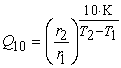(1)

Herein,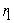and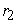stand for reaction rates at temperatures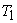and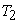in Kelvin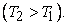Even if this rule is only a rough approximation, the determination of the dimensionless temperature coefficient Q10 has proven its worth for the estimation of thermal dependencies in numerous biological processes. To derive this rule of thumb for chemical reactions based on experiments, various physical parameters can be utilized. Typical examples for digitally recorded changes of physical quantities in chemical kinetics are temperature, mass, conductivity, light absorption, and pH.

2.2. Heat Tinting: Catalytic Oxidation of Tartaric Acid with Hydrogen Peroxide

The oxidation of tartaric acid with hydrogen peroxide is a popular and well-established experiment in chemical education that can be used in a variety of kinetics demonstrations (cf., e.g., 6, 7, 8, 9). Mostly, this reaction is utilized to demonstrate the effect of a catalyst, the role of an activated complex or the regeneration of a catalyst. Without a catalyst, the activation barrier for the oxidation of tartaric acid reaction is quite high and the reaction proceeds very slow at room temperature. The addition of cobalt(II) chloride hexahydrate as a catalyst speeds up the reaction considerably. The overall reaction equation can be written as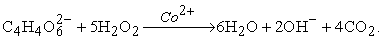The reaction progress is evidenced by color changes accompanied by gas evolution and a rise in temperature due to the strongly exothermic nature of the reaction. Therefore, it can be followed by light absorption, gravimetrically, or by temperature measurements.

2.2.1. Experimental Procedure

Based on the procedure given in 8, 3 g of potassium sodium tartrate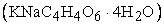were dissolved in 15 ml distilled water at 30 °C on a hot plate in a 250 ml beaker. In the meantime, 20 ml of 30% hydrogen peroxide were heated up to 30 °C in a 100 ml beaker. A NiCr-Ni-thermocouple (connected with a digital data logging system) was immersed into the tartrate solution and 10 ml of the hydrogen peroxide solution were added. The temperature measurement was started and 100 mg of cobalt(II) chloride (CoCl2 ∙ 6 H2O) were added after one minute. A series of such experiments was conducted at intended initial temperatures of 30 °C, 40 °C, 50 °C, 60 °C, and 70 °C employing a CASSY® measuring device from LD Didactic.

2.2.2. Results and Discussion

In different states of the reaction, typical color changes were observed. Initially, the addition of cobalt(II) chloride gives the solution a pink color. The color changes to green due to an intermediate cobalt-tartrate complex (activated Co(III) complex). In this stage, the reaction becomes most vigorous, as indicated by intensive gas evolution. Finally, the regeneration of the catalyst is indicated by a return of its color to the original pink after an intermediate brown phase. The color changes during the reaction process are shown in Figure 1.

• Figure 1. Color changes during the oxidation of tartaric acid (a: after adding the catalyst, b: green activated complex, c and d: catalyst regeneration)

It might seem paradoxical at first to record the heat tone of a chemical reaction to derive the reaction rate temperature dependency. However, due to the strongly exothermic reaction, relative reaction rates can be determined by measuring the time elapsed between the catalyst addition and the temperature maximum. Therefore, the temperature changes were measured during the reaction and were monitored for different initial temperatures. The results are presented in Figure 2.

As is evident from the data in Figure 2, an increase of temperature of about 10 °C reduces the reaction time by approximately 50%. For example, increasing the initial temperature from 50 °C to 60 °C yields a Q10 temperature coefficient of 1.92, which is in good agreement with the van‘t Hoff rule. Measurements at temperatures above 70 °C are impractical due to strong gas evolution causing foam to flow over the beaker sides.

• Figure 2. Temperature profiles for the catalytic oxidation of tartaric acid at different initial temperatures (data starting with adding the catalyst)
• Figure 3. Comparison of thermal images during the oxidation of tartaric acid with different initial temperatures (left column: initial state while adding the catalyst, middle column: while running the reaction, and right column: at the temperature maximum)

As an alternative to the traditional thermocouple, the temperature change of the reaction can also be recorded contactless with an infrared thermal imaging camera. To guarantee appropriate recording conditions, the chemical scale must be doubled, which may prove to be disadvantageous for practical courses. The results recorded with a thermal imaging system placed 10 cm above the beaker rim are presented in Figure 3 for initial temperatures of 30 °C and 65 °C. Following the results obtained with the thermocouple, one would expect for an initial temperature difference of 35 °C an increase of the reaction rate by a factor of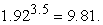The factor obtained from thermal images for the temperature maxima ofis in reasonable agreement with the thermocouple value. Due to the larger amount of chemicals needed for infrared thermal imaging, investigations at initial temperatures above 65 °C are not recommended. However, if the absolute temperature values are not the focus of interest, thermography is an excellent example of a visualization technique that can be used with a simple experimental setup - also in practical courses.

2.3. Light Absorption: The Oscillating Briggs-Rauscher Reaction

Chemical reactions with color changes are well suited for demonstration purposes. Furthermore, such reactions are easy to track photometrically because changes in the extinction can be directly related to concentration changes. One of the most popular oscillating reactions in lectures on chemical kinetics is the so-called Briggs-Rauscher reaction (cf., e.g., 10, 11, 12). Although its mechanism is quite complex, including non-radical and radical processes, the overall reaction equation can be written as: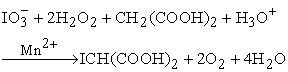To study the temperature dependency of the Briggs-Rauscher reaction, initial aqueous solutions containing hydrogen peroxide, malonic acid, manganese sulfate (as catalyst), starch (to indicate the iodine-starch-complex), potassium iodate, and sulfuric acid were prepared following the procedure in 13. After mixing the initial solutions, the observed periodic color changes from amber to very dark blue were followed photometrically with an immersion photometer at a wavelength of 455 nm. The results for initial temperatures of 28 °C, 38 °C, and 48 °C (observed with a thermocouple) are presented in Figure 4. As can be seen, the oscillation times between the “blue phases” (transmission minima) decrease with increasing temperature. In the range of constant oscillations, averaged oscillation frequencies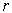can be observed from the transmission curves at different temperatures. This procedure yields Q10 temperature coefficients-summarized in Table 1 - which are in reasonable agreement with van‘t Hoff rule.

• Figure 4. Transmission curves at 455 nm of the oscillating Briggs-Rauscher reaction at different temperatures

### 3. Experiments to Exploit and to Derive the Arrhenius Equation

3.1. Light Absorption: Discoloration of Triphenyl Methane Dyes

The traditional way to teach the effect of temperature on reaction rates in chemical education on a more sophisticated basis usually follows a deductive approach based primarily on theoretical considerations.

Initially, in lectures on physical chemistry, the basics of collision theory are introduced, which state that the rate of a chemical reaction is proportional to the number of collisions between reacting molecules. However, a collision results in a chemical reaction only if a certain threshold energy is exceeded and if the molecules collide with the correct orientation. These requirements are then summarized by the Arrhenius equation: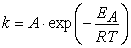(2)

with k being the reaction rate constant, R the universal gas constant, and T the absolute temperature. The quantity A is called the frequency factor and can be interpreted as the maximum reaction rate constant at infinitely large temperature, whereas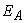is the activation energy of the reaction. At room temperature, the activation energycorresponds approximately to the minimum energy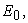which molecules must possess in order to react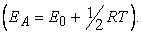In a second step, the so-called Arrhenius plot is frequently used in practical courses to determine the parameters A andin experiments for appropriate chemical reactions. Therefore, the Arrhenius equation is “linearized” by logarithmizing Eq. 2, giving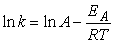(3)

In a slightly rearranged form, the equation becomes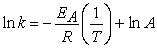(4)

and the plot of ln k vs. 1/T yields a linear graph. The values of the reaction rate constant are determined experimentally at different temperatures. The slope of this graph is equal to the negative activation energy divided by the gas constant. The yintercept of this function yields the value for ln A.

3.1.1. Discoloration of Crystal Violet

A well-established example for discoloration reactions is the discoloration of crystal violet in an alkaline medium (cf., e.g., 1, 14, 15, 16). Based on the procedure given in 17, 25 ml of 0.1 M sodium hydroxide solution were added to 25 ml 5 ∙105 M crystal violet solution. The absorbance of the discoloration reaction was recorded with an immersion photometer at a wavelength of 558 nm (λmax of crystal violet is about 587 nm 14) at initial temperatures of 20 °C, 30 °C, 40 °C, 50 °C, 60 °C, and 70 °C.

Crystal violet is a cationic triphenyl methane dye (Figure 6). The violet color of its solution in the pH range from weakly acidic to weakly basic originates from the extended conjugated π-system as the key feature of the dye. Once sodium hydroxide solution is added, the nucleophilic hydroxide ions attack the electrophilic central carbon to produce the colorless carbinol form of the dye due to the disruption of the conjugated system. Because of the large excess of hydroxide ions, described under the experimental conditions above, the reaction is of pseudo-first order. By using Lambert-Beer-Bouguer Law, the integrated rate law of pseudo-first order yields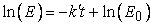(5)

where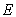and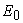is the absorbance at 558 nm at the time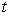and at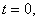respectively. According to Eq. 5, the effective reaction rate constant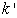can be obtained from the negative slope of the linear graph at different temperatures. The determined values are summarized in Table 2.

Lastly, the calculated reaction rate constants can be used to determine the activation energy and the frequency factor. The corresponding Arrhenius plot is presented in Figure 5. The activation energy of EA = 55.2 kJ/mol calculated from the slope of the graph is within the range of published data for the discoloration of crystal violet between 64 kJ/mol 1 and 47.4 kJ/mol 17.

• Figure 5. Arrhenius plot for the reaction of crystal violet with sodium hydroxide
• Figure 6. Nucleophilic discoloration reactions of crystal violet (a) and brilliant blue FCF (b)

3.1.2. Discoloration of Brilliant Blue FCF

Food colorings may be used as an alternative to connect kinetic studies closer to students’ everyday lives. The discoloration of brilliant blue FCF (cf., Figure 6) with sodium hypochlorite may serve as an example. Brilliant blue FCF - known as E133 - is widely used by food manufacturers. The pseudo-first order reaction of the discoloration of the food coloring is similar to the reaction of crystal violet. However, a key advantage is the harmlessness of brilliant blue FCF compared to the presumed carcinogen crystal violet. This food coloring is well suited to spectroscopic studies due to its extraordinary large attenuation coefficient of ε = 10066 l∙mol-1∙cm-1 at λmax of 638 nm 18.

To make this experiment suitable for classrooms and practical courses, a low-cost setup has been chosen. The basis of this setup is a 3D-printed insulation photometer working with light emitting diodes (LED) as a light source, as well as a detector (cf. 18). The amount of light is proportional to the voltage of the induced photocurrent in the detector LED. A low-cost voltage-divider in combination with an operational amplifier was used to measure this voltage. All electronic parts were connected with an Arduino Uno microcontroller to provide a compact setup and the ability to record the measured data. In order to obtain a user-friendly working low-cost device, an additional graphical user interface (GUI) was designed with the software MATLAB. This allows learners to both control the microcontroller, and evaluate the measured data immediately. The complete setup of the microcontroller is shown in Figure 7.

• Figure 7. Low-cost immersion photometer setup connected to an Arduino Uno microcontroller (a) and data logging GUI designed with MATLAB (b)

The experimental procedure is similar to the nucleophilic discoloration reaction of crystal violet, except that a 6% solution of sodium hypochlorite was used as an oxidant. The discoloration reaction would also be successful with sodium hydroxide, but the sulfonate groups of brilliant blue FCF inhibit the nucleophilic attack, resulting in a long reaction time (about 24 h), which would not be feasible for practical courses. Consequently, 5 ml of a 6% solution of sodium hypochlorite were added to 120 ml of 2∙105 M solution of brilliant blue FCF. The absorbance of the discoloration reaction was recorded with the previously described low-cost photometer near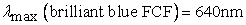18 at initial temperatures of 22 °C, 40 °C and 60 °C.

The reaction rate constants at the various temperatures, which can be determined in analogy to the procedure described in section 3.1.1., are summarized in Table 3.

Finally, an Arrhenius plot based on the reaction rate constants can be plotted. The calculated activation energy EA = 57.5 kJ/mol of the reaction, which is represented by the slope of the Arrhenius plot, is plausible for the examined reaction (cf., e.g., 19).

3.2. Loss of Weight: Decomposition of Marble

The inductive approach to derive the Arrhenius equation experimentally is perceptibly more difficult. Initially, experimental data must be recorded for a chemical reaction with a noticeable temperature dependency of the reaction rate. A well-suited example for this is the decomposition of marble with hydrochloric acid (cf., e.g., 1, 20, 21).

The chemical reaction behind the decomposition of marble or calcite with the general composition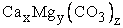can be written in simplified form as: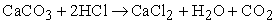Thus, with known amounts of marble and hydrochloric acid, the loss of weight due to the carbon dioxide generation in an open system can be measured gravimetrically. The sub-steps of the whole process - diffusion of reactants to, and of products from the marble surface, decomposition of marble, and decay of carbonic acid to carbon dioxide and water - are more complex. However, at the beginning, the reaction obeys the rate law of a pseudo-zero order reaction on the condition that large pieces of marble as well as an excess of hydrochloric acid are used in the experiment. Under these conditions, the rate-determining step is the decomposition reaction of marble.

3.2.1. Experimental Procedure

Initially, 80 ml hydrochloric acid (37%) were dissolved in 80 ml distilled water. 25 ml portions (V0) of this semi-concentrated acid solution (c0 = 6 mol/l) were transferred to 100 ml beakers and brought to the various initial temperatures (10 °C, 25 °C, 40 °C, 50 °C, and 65 °C). Simultaneously, the pieces of marble (about 10 g each, approx. equal size) were also brought to the required temperatures. Finally, the beakers were placed on a scale (connected with a digital data logging system), the marble pieces were added, and the measurements were started in a thermally insulated environment.

3.2.2. Results and Discussion

After the start of the reaction, a strong gas evolution accompanied by a loss of weight were observed. The observed weight losses can be converted into actual hydrochloric acid concentrations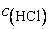by using Eq. 6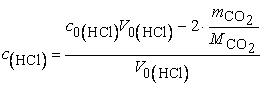(6)

The resulting concentration changes plotted against time are presented in Figure 8. As can be seen, at the beginning (first 60 seconds) the reaction is of pseudo-zero order at each temperature, and the reaction rate constants k’ can be derived from the slopes of the linear subgraphs following the integrated rate law of a pseudo-zero order reaction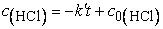(7)
• Figure 8. The concentration changes of HCl plotted against time at different temperatures

In analogy to Figure 5, the plot of ln k’ vs. 1/T yields an activation energy of EA = 24.4 kJ/mol for the decomposition of marble, in good agreement with the value of EA = 19 ± 4 kJ/mol reported by Alkattan et al. 22. Beyond an elapsed time of 120 seconds, the reaction follows a first-order rate law. The evaluation of experimental data on the basis of a first order reaction yields an activation energy of EA = 22.3 kJ/mol. However, the considerably greater evolution of heat over extended reaction time results in a noticeably larger error in the Arrhenius plot.

• Figure 9. Plot of the reaction rate constants against the absolute temperature T for the decomposition of marble
• Figure 10. Temperature dependency of the reaction rate constant

In contrast, the inductive way of deriving a mathematical formula for the Arrhenius equation from experimental data starts with the graphical plot of reaction rate constants k’ against the accompanying absolute temperatures T. This plot is shown in Figure 9.

It can be seen that the correlation is exponential rather than linear. In principle, over the whole temperature range the wanted mathematical function must fulfill the following boundary conditions:

(i) From collision theory, it is evident that at very low temperatures near 0 K the reactant particles are more or less frozen, the collisions are therefore too gentle and consequently, the rate constant should reach a value of zero in the very low temperature range.

(ii) At moderate temperatures, as implemented in the experiments, the dependency k’(T) should follow an exponential function (Figure 9).

(iii) At very high temperatures, the rate constant increases more slowly and must converge to a limit because the total number of particles is limited and the duration of each collision is greater than zero.

The resulting course of the graph over the whole temperature range is illustrated in Figure 10.

To model the course of the shown graph mathematically, for area (ii) a general exponential function of the form: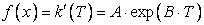(8)

can be postulated, whereas for area (iii) the function: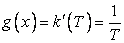(9)

describes the graph progression best. The composition of both functions yields the resulting composite function: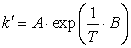(10)

In practical courses for students, one viable option to visualize the graph’s shape is to use the graphing calculator GeoGebra 23. With this freeware tool, the perfect match between predicted (Figure 10) and calculated graph courses can be observed interactively by adjusting values of variables with sliders. As can be seen in Figure 11, the perfect match is achieved with a negative exponent with the resulting function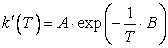(11)
• Figure 11. Exemplary graph of the required mathematical function in the positive (physically meaningful) range of absolute temperatures plotted with GeoGebra

The physical meaning of the negative exponent is still worthy of discussion for students without a thermodynamic background. However, for the physical interpretation of the parameter A (frequency factor) it is obvious that for T→∞ the parameter A converges to the maximum reaction rate constant k’. This means that for T→∞ all reactant particles have the necessary minimum energy and all collisions are successful. For lower temperatures, the exponential term then specifies the proportion of energy-rich particles, and for this reason must include the activation energy within the parameter B. With the decreasing minimum energy, the number of reactive particles increases as a consequence of the Maxwell-Boltzmann distribution, and consequently, the value of the reaction rate constant rises. Mathematically, this leads to the conclusion that the activation energy EA must be part of the numerator in the exponential term of Eq. 11. In a final step, a further proportionality factor in the denominator is necessary to ensure that the complete exponent in Eq. 11 is physically dimensionless. Therefore, a proportionality factor with the unit J/mol∙ K is required, which corresponds exactly to the universal gas constant leading to the factor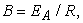and thus to the complete Arrhenius equation (Eq. 2). Although this mathematical modeling approach is of course not equivalent to a physicochemical proof, it is a suitable way to derive the Arrhenius equation from experimental data in laboratory courses.

### 4. Summary and Conclusions

In this contribution, basic kinetic experiments have been developed to give learners phenomenological access to the temperature dependency of the reaction rate in chemical reactions. A deductive approach to the basic principles of kinetics is often used in lectures, or once learners are already familiar with essentials of chemical thermodynamics. In terms of a constructivist and experimentally orientated education, an inductive approach could be more promising.

An initial simple correlation between temperature and reaction rate is represented in the van‘t Hoff rule. This rule can be derived inductively from the catalytic oxidation reaction of tartaric acid at different temperatures or by the more complex oscillating Briggs-Rauscher reaction. Learners can follow the progresses of both reactions visually due to their characteristic color changes. The use of an infrared thermal imaging camera reinforces this visual component of the experiments.

The key results of chemical kinetic studies are summarized by the Arrhenius equation. An established deductive approach to the equation is the discoloration reaction of crystal violet with sodium hydroxide at different temperatures. Thereby, important kinetic parameters such as the activation energy can be determined by combining the Arrhenius equation with the Lambert-Beer-Bouguer law. A setup with a low-cost immersion photometer paired with an Arduino microcontroller can make discoloration experiments accessible for practical courses with low budgets. Thereby, the oxidation of the food coloring brilliant blue FCF can additionally connect kinetic studies to learners’ everyday lives.

The inductive way of deriving the Arrhenius equation from experimental data is mainly based on a mathematic modeling process. Of course, the derivation follows a purely phenomenological approach. One needs to keep in mind that it does not follow a strict physico-chemical justifiable reason. However, this method may lead to improved understanding for abstract kinetic parameters such as the frequency factor and their physical interpretation.

### Acknowledgements

The authors thankfully acknowledge Wolfgang Obert for the technical support.

This publication was funded by the German Research Foundation (DFG) and the University of Würzburg in the funding program Open Access Publishing.

### References

  Habekost, A., Einführung in die Reaktionskinetik: Theorie und Praxis, Hildesheim, Franzbecker, 2010. In article  Seoud, O. A. E., Baader, W. J., and Bastos, E. L., “Practical Chemical Kinetics in Solution,” in Encyclopedia of Physical Organic Chemistry, American Cancer Society, 2016, 1-68. In article View Article  Jansen, W. and Matuschek-Wilken, C., “Das historisch problemorientierte Unterrichtsverfahren - Geschichte der Chemie im Chemieunterricht,” in Konkrete Fachdidaktik Chemie, 3rd ed., , P. Pfeifer, B. Lutz, and Bader, Hans Joachim, Eds. München, Oldenbourg, 2002. In article  Arrhenius, S., “Über die Reaktionsgeschwindigkeit bei der Inversion von Rohrzucker durch Säuren,” Zeitschrift für Physikalische Chemie, 4U(1), 226-248, 1889. In article View Article  Harel, I. and Papert, S., Constructionism, Norwood, Ablex Publishing, 1991. In article  Ruda, P. T., “A Versatile kinetics demonstration,” Journal of Chemical Education, 55(10), 652, Oct. 1978. In article View Article  Wiechoczek, D. and Blume, R., “Die durch Cobalt katalysierte Oxidation von Weinsäure,” 04-Jan-2005. [Online]. Available: http://www.chemieunterricht.de/dc2/katalyse/vkat-008.htm. [Accessed: 18-Dec-2019]. In article  LD Didactic, “Katalytische Oxidation von Weinsäure mit Wasserstoffperoxid.” [Online]. Available: https://www.ld-didactic.de/documents/de-DE/EXP/C/C4/C4111_d.pdf. [Accessed: 18-Dec-2019]. In article  Wright, S. W. and Silverstein, T. P., “Improvements upon the ‘Colorful Cobalt Catalysis’ Demonstration and Evidence for the Presence of an Autocatalytic Mechanism,” Journal of Chemical Education, 87(10), 1064-1069, Oct. 2010. In article View Article  Briggs, T. S. and Rauscher, W. C., “An oscillating iodine clock,” Journal of Chemical Education, 50(7), 496, Jul. 1973. In article View Article  Brice, L. K., “Rossini, William Tell and the iodine clock reaction: A lecture demonstration,” Journal of Chemical Education, 57(2), 152, Feb. 1980. In article View Article  Creary, X. and Morris, K. M., “A New Twist on the Iodine Clock Reaction: Determining the Order of a Reaction,” Journal of Chemical Education, 76(4), 530, Apr. 1999. In article View Article  Kreißl, F. R. and Krätz, O., Feuer und Flamme, Schall und Rauch, Weinheim, Wiley-VCH, 1999. In article  Felix, L. D., “Kinetic Study of the Discoloration of Crystal Violet Dye in Sodium Hydroxide Medium,” Journal of Chemistry and Applied Chemical Engineering, 2018, May 2018. In article  Kappenberg, F., “Reaktion von Kristallviolett mit Natronlauge,” Jun-2009. [Online]. Available: http://www.kappenberg.com/experiments/foto/pdf/i07.pdf. In article  Kazmierczak, N. and Vander Griend, D. A., “Improving Student Results in the Crystal Violet Chemical Kinetics Experiment,” Journal of Chemical Education, 94(1), 61-66, Jan. 2017. In article View Article  Ralle, B. et al., Handbuch der experimentellen Chemie. Sekundarbereich II / Kinetik, Katalyse, Gleichgewicht, Köln, Aulis Verlag, 2004. In article  Gräb, P. and Geidel, E., “Spectroscopic Studies of Food Colorings,” World Journal of Chemical Education, 7(2), 136-144, Apr. 2019. In article View Article  Nadupalli, S., Dasireddy, V., Koorbanally, N., and Jonnalagadda, S., “Kinetics and Mechanism of the Oxidation of Coomassie Brilliant Blue-R Dye by Hypochlorite and Role of Acid Therein,” South African Journal of Chemistry, 68, 85-92, 2015. In article View Article  Steiner, D. and Lehnerer, M., “Reaktionsgeschwindigkeiten mit einer Waage aufgezeichnet,” Naturwissenschaften im Unterricht Chemie, 11, 34-36, 1992. In article  Baedecker, P. A. and Reddy, M. M., “The Erosion of Carbonate Stone by Acid Rain: Laboratory and Field Investigations,” Journal of Chemical Education, 70(2), 104, Feb. 1993. In article View Article  Alkattan, M., Oelkers, E. H., Dandurand, J.-L., and Schott, J., “An experimental study of calcite and limestone dissolution rates as a function of pH from -1 to 3 and temperature from 25 to 80°C,” Chemical Geology, 151(1-4), 199-214, Oct. 1998. In article View Article  International GeoGebra Institute, “GeoGebra.” [Online]. Available: https://www.geogebra.org/. [Accessed: 13-Feb-2020]. In articleThis work is licensed under a Creative Commons Attribution 4.0 International License. To view a copy of this license, visit http://creativecommons.org/licenses/by/4.0/